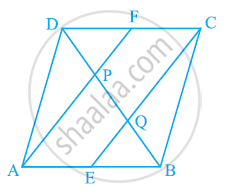# Find the values of k for each of the following quadratic equations, so that they have two equal roots. 2x^2 + kx + 3 = 0 - Mathematics

In a parallelogrFind the values of k for each of the following quadratic equations, so that they have two equal roots. 2x2 + kx + 3 = 0#### Solution

ABCD is a parallelogram.

∴ AB || CD

And hence, AE || FC

Again, AB = CD (Opposite sides of parallelogram ABCD)

1/2AB =1/2CD

AE = FC (E and F are mid-points of side AB and CD)

In quadrilateral AECF, one pair of opposite sides (AE and CF) is parallel and equal to each other. Therefore, AECF is a parallelogram.

⇒ AF || EC (Opposite sides of a parallelogram)

In ΔDQC, F is the mid-point of side DC and FP || CQ (as AF || EC). Therefore, by using the converse of mid-point theorem, it can be said that P is the mid-point of DQ.

⇒ DP = PQ ... (1)

Similarly, in ΔAPB, E is the mid-point of side AB and EQ || AP (as AF || EC). Therefore, by using the converse of mid-point theorem, it can be said that

Q is the mid-point of PB.

⇒ PQ = QB ... (2)

From equations (1) and (2),

DP = PQ = BQ

Hence, the line segments AF and EC trisect the diagonal BD.

Concept: The Mid-point Theorem
Is there an error in this question or solution?

#### APPEARS IN

NCERT Class 9 Maths